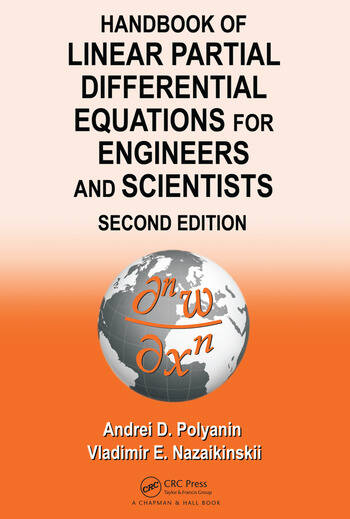# Handbook of Linear Partial Differential Equations for Engineers and Scientists

## 2nd Edition

Andrei D. Polyanin, Vladimir E. Nazaikinskii

Chapman and Hall/CRC
Published January 15, 2016
Reference - 1609 Pages - 28 B/W Illustrations
ISBN 9781466581456 - CAT# K18874

was \$215.00

USD\$172.00

SAVE ~\$43.00

FREE Standard Shipping!

## Preview

### Summary

• Includes nearly 4,000 linear partial differential equations (PDEs) with solutions
• Presents solutions of numerous problems relevant to heat and mass transfer, wave theory, hydrodynamics, aerodynamics, elasticity, acoustics, electrodynamics, diffraction theory, quantum mechanics, chemical engineering sciences, electrical engineering, and other fields
• Outlines basic methods for solving various problems in science and engineering
• Contains much more linear equations, problems, and solutions than any other book currently available
• Provides a database of test problems for numerical and approximate analytical methods for solving linear PDEs and systems of coupled PDEs

New to the Second Edition

• More than 700 pages with 1,500+ new first-, second-, third-, fourth-, and higher-order linear equations with solutions
• Systems of coupled PDEs with solutions
• Some analytical methods, including decomposition methods and their applications
• Symbolic and numerical methods for solving linear PDEs with Maple, Mathematica, and MATLAB®
• Many new problems, illustrative examples, tables, and figures

To accommodate different mathematical backgrounds, the authors avoid wherever possible the use of special terminology, outline some of the methods in a schematic, simplified manner, and arrange the material in increasing order of complexity.